Home | | Maths 12th Std | Series Expansions: MaclaurinŌĆÖs and TaylorŌĆÖs Series

# Series Expansions: MaclaurinŌĆÖs and TaylorŌĆÖs Series

TaylorŌĆÖs series and Maclaurin's series expansion of a function which are infinitely differentiable.

Series Expansions

TaylorŌĆÖs series and Maclaurin's series expansion of a function which are infinitely differentiable.

Theorem 7.5

## (a) TaylorŌĆÖs Series

Let f ( x) be a function infinitely differentiable at x = a . Then f ( x) can be expanded as a series, in an interval ( x ŌłÆ a, x + a) , of the form(b) MaclaurinŌĆÖs series

If a = 0 , the expansion takes the form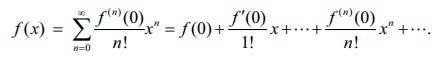## Proof

The series expansion of f ( x) , in powers of ( x ŌłÆ a) , be given bySubstituting x = a gives A0 = f (a) . Differentiation of (7) gives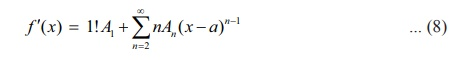Substituting x = a gives A1 = f ŌĆ▓(a) . Differentiation of (8) givesSubstituting x = a gives A2 = f ŌĆ▓ŌĆ▓(a) / 2!. Differentiation of (9) givesDifferentiation of (10) (k ŌłÆ 3) times gives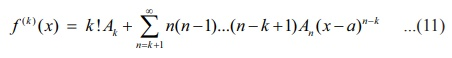Substituting x = a gives

Ak f (k)(a) / k!which completes the proof of the theorem.

In order to expand a function around a point say x = a , equivalently in powers of ( x ŌłÆ a) we need to differentiate the given function as many times as the required powers and evaluate at x = a . This will give the value for the coefficients of the required powers of ( x ŌłÆ a) .

Example 7.30

Expand log(1+ x) as a MaclaurinŌĆÖs series upto 4 non-zero terms for ŌĆō1 < x Ōēż 1.

Solution

Let f (x) = log(1+ x) , then the Maclaurin series of f (x) is f (x)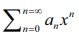, where,various derivatives of the function f (x) evaluated at x = 0 are given below: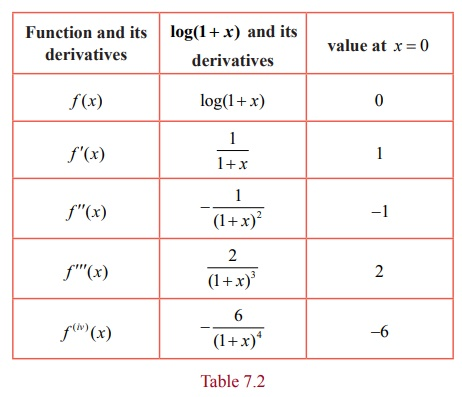Substituting the values and on simplification we get the required expansion of the function given by,### Example 7.31

Expand tan x in ascending powers of x upto 5th power for ŌĆō ŽĆ/2 < x < ŽĆ/2.

Solution

Let f (x) = tan x . Then the Mclaurin series of f ( x) isVarious derivatives of the function f ( x) evaluated at x = 0 are given below:

Now,

f ŌĆ▓(x) = d/dx (tan x ) = sec2(x)

fŌĆÖŌĆ▓(x) = d/dx (sec2 ( x )) = 2 sec x Ōŗģ sec x Ōŗģ tan x = 2 sec2x Ōŗģ tan x

f ŌĆ▓ŌĆ▓ŌĆ▓(x) = d/dx (2sec2 ( x ). tan x) = 2 sec2(x ) Ōŗģ sec2x + tan x Ōŗģ 4 sec x Ōŗģ sec x Ōŗģ tan x

= 2 sec4 x + 4 sec2 x Ōŗģ tan2 x

f (iv)(x) = 8sec3 x Ōŗģ sec x Ōŗģ tan x + 4 sec2 x Ōŗģ 2 tan x Ōŗģ sec2 x + 8sec x Ōŗģ sec x Ōŗģ tan x Ōŗģ tan2 x

= 16 sec4 x tan x + 8sec2 x Ōŗģ tan3 x

f(v)( x) = 16 sec4 x Ōŗģ sec2 x + 64 sec3 x Ōŗģ sec x Ōŗģ tan x Ōŗģ tan x + 8sec2 x Ōŗģ 3 tan2 x Ōŗģ sec2 x +16 sec x Ōŗģ sec x Ōŗģ tan x Ōŗģ tan3 x

= 16 sec6 x + 88sec4 x Ōŗģ tan2 x + 16 sec2 x Ōŗģ tan4 x .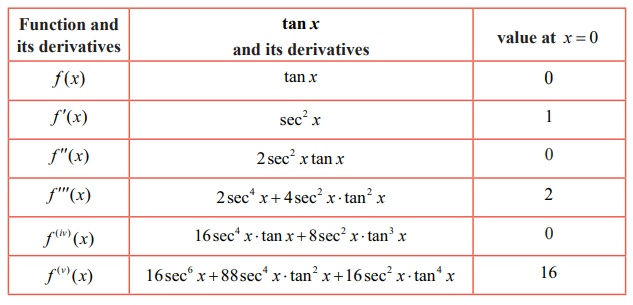Substituting the values and on simplification we get the required expansion of the function as

tan x = x + 1/3 x3 + 2/15 x5 + .. ŌĆ” ;       ŌłÆ ŽĆ/2 < x < ŽĆ/2

### Example 7.32

Write the Taylor series expansion of 1/ x about x = 2 by finding the first three non-zero terms.

Solution

Let f (x) = 1/x, then the Taylor series of f ( x) isVarious derivatives of the function  f ( x) evaluated at x = 2 are given below.Substituting these values, we get the required expansion of the function asTags : Differential Calculus | Mathematics , 12th Maths : UNIT 7 : Applications of Differential Calculus
Study Material, Lecturing Notes, Assignment, Reference, Wiki description explanation, brief detail
12th Maths : UNIT 7 : Applications of Differential Calculus : Series Expansions: MaclaurinŌĆÖs and TaylorŌĆÖs Series | Differential Calculus | Mathematics